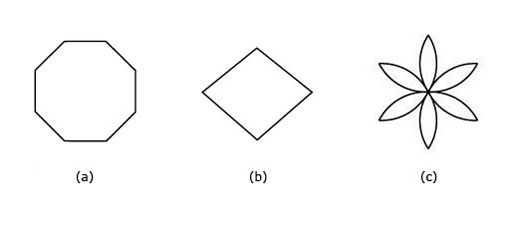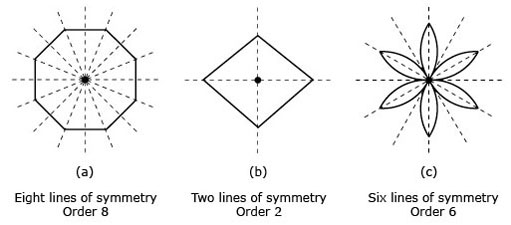Science, Maths & Technology

### Become an OU studentGeometry

Start this free course now. Just create an account and sign in. Enrol and complete the course for a free statement of participation or digital badge if available.

# Try some yourself

## Question 1

Draw a line of symmetry on each of the shapes below.

Each of the shapes has only one line of symmetry, so these are the only possible answers.

## Question 2

Mark all of the lines of symmetry on these shapes. For each shape, state the total number of lines of symmetry.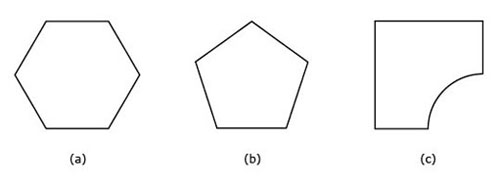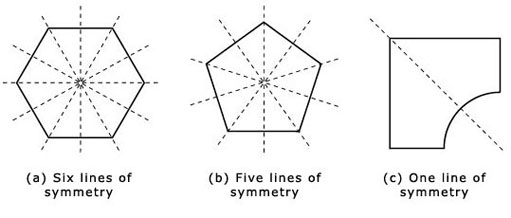## Question 3

• (a) The dartboard has rotational symmetry.

• (b) The letter Z has rotational symmetry.

• (c) The letter K does not have rotational symmetry.

## Question 4

Mark the centre of rotation on each of the shapes below. For each, state the order of rotational symmetry.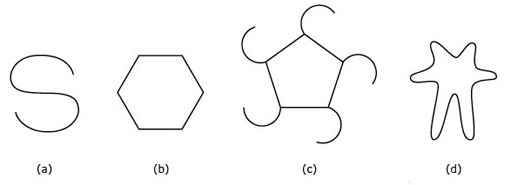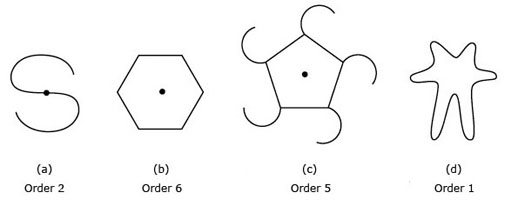Notice that (d) has no rotational symmetry and no centre of rotation.

## Question 5

Describe the symmetry of each of these shapes. Mark all the lines of symmetry in each case. Also mark the centre of rotation, and state the order of rotational symmetry.